﻿ Implicit Analysis of Al-Mn Alloy Corrosion Rate Dependence on Its Pre-Installed Weight and Exposure Time in Atmosphere Environment

### Implicit Analysis of Al-Mn Alloy Corrosion Rate Dependence on Its Pre-Installed Weight and Exposure ...

C. Nwoye, E. O. Obidiegwu, N. E. Idenyi

## Implicit Analysis of Al-Mn Alloy Corrosion Rate Dependence on Its Pre-Installed Weight and Exposure Time in Atmosphere Environment

C. Nwoye1,, E. O. Obidiegwu2, N. E. Idenyi3

1Department of Metallurgical and Materials Engineering, Nnamdi Azikiwe University, Awka, Nigeria

2Department of Metallurgical and Materials Engineering, University of Lagos, Akoka, Nigeria

3Department of Industrial Physics, Ebonyi State University, Abakiliki, Nigeria

### Abstract

An implicit analysis of the Al-Mn corrosion rate dependence on the pre-installed alloy weight and exposure time in atmosphere environment was carried out. Surface structural analysis of corroded and uncorroded Al-Mn alloys were carried out to evaluate the grain boundary morphology. The response coefficient of the alloy corrosion rate to the combined influence of pre-installed alloy weight ϑ and exposure time ɤ was evaluated to ascertain the viability and reliability of the highlighted dependence. Surface structural analysis of the corroded alloy revealed in all cases widely distributed oxide film of the alloy in whitish form. A two-factorial empirical model was derived, validated and used for the analysis and evaluation. The validity of the model; ζ = Log -1(2.4908 (ϑ/ɤ) - 4.3059 (ϑ/ɤ)2 - 2.5941) was rooted on the core model expression Log ζ + 2.5941 = 2.4908 (ϑ/ɤ) - 4.3059 (ϑ/ɤ)2 where both sides of the expression are correspondingly approximately equal. Results generated using regression model showed trend of data point distribution similar to those from experiment and derived model. Evaluations from generated results indicated that the corrosion penetration depth as obtained from experiment, derived model & regression model were 1.394 x 10-5, 1.886 x 10-5 & 1.394 x 10-5 mm respectively. Standard errors incurred in predicting the corrosion rate for each value of the pre-installed alloy weight and exposure time considered as obtained from experiment, derived model & regression model were 8.21 x 10-5, 9.91 x 10-5 & 2.02 x 10-5 % and 5.46 x 10-5, 1.4 x 10-4 & 5.18 x 10-5 % respectively. Deviational analysis indicates that the maximum deviation of model-predicted corrosion rate from the experimental results is less than 19%. This translated into over 80% operational confidence and response level for the derived model as well as over 0.8 response coefficient of corrosion rate to the collective operational contributions of pre-installed alloy weight and exposure time in the atmosphere environment.

• C. Nwoye, E. O. Obidiegwu, N. E. Idenyi. Implicit Analysis of Al-Mn Alloy Corrosion Rate Dependence on Its Pre-Installed Weight and Exposure Time in Atmosphere Environment. International Journal of Materials Lifetime. Vol. 2, No. 1, 2015, pp 22-29. http://pubs.sciepub.com/ijml/2/1/4
• Nwoye, C., E. O. Obidiegwu, and N. E. Idenyi. "Implicit Analysis of Al-Mn Alloy Corrosion Rate Dependence on Its Pre-Installed Weight and Exposure Time in Atmosphere Environment." International Journal of Materials Lifetime 2.1 (2015): 22-29.
• Nwoye, C. , Obidiegwu, E. O. , & Idenyi, N. E. (2015). Implicit Analysis of Al-Mn Alloy Corrosion Rate Dependence on Its Pre-Installed Weight and Exposure Time in Atmosphere Environment. International Journal of Materials Lifetime, 2(1), 22-29.
• Nwoye, C., E. O. Obidiegwu, and N. E. Idenyi. "Implicit Analysis of Al-Mn Alloy Corrosion Rate Dependence on Its Pre-Installed Weight and Exposure Time in Atmosphere Environment." International Journal of Materials Lifetime 2, no. 1 (2015): 22-29.

 Import into BibTeX Import into EndNote Import into RefMan Import into RefWorks

12345
Prev Next

### 1. Introduction

The high level and incessant in-service failures of metallic parts and components caused by corrosion and its attendant consequences have raised an unavoidable need for intensive and extensive research & development aimed at repositioning molten technological advancement and its full utilization. Furthermore, there is a corresponding need for an effective and efficient means of protecting metallic and alloy based structures from corrosion attack. It is strongly believed that the study of corrosion, its mode of attack, its testing, evaluation and monitoring are very vital in today’s processes so as to achieve a high level of quality and safety assurance in the selection and usage of metals and alloys.

Research  has shown that metallic corrosion is basically electrochemical, involving both oxidation and reduction reactions. Oxidation is the loss of the metal atom’s valence electrons; the resulting metal ions may either go into the corroding solution or form an insoluble compound. The scientist posited that these electrons are transferred to at least one other chemical species during reduction. The researcher further reveal that the character of the corrosion environment dictates which of several possible reduction reactions will occur.

Studies [2, 3] have revealed that a number of metals and alloys passivate, or lose their chemical reactivity, under some environmental circumstances. This phenomenon basically involve the formation of a thin protective oxide film which varies with atmospheric conditions, type and amount of pollutants as well as wet-dry cycle, the chemical composition and metallurgical history of the metals or alloys and physico-chemical properties of coating. Stainless steels and aluminum alloys exhibit this type of behavior. The alloy’s S-shaped electrochemical potential-versus-log current density curve  ideally explains this active-to-passive behavior. Furthermore, the intersections with reduction polarization curves in active and passive regions correspond, respectively, to high and low corrosion rates.

Aluminum and its alloys are highly corrosion resistant in many environments because it passivates. If damaged, the protective film normally reforms very rapidly. However, report  has shown that a change in the character of the environment (e.g., alteration in the concentration of the active corrosive species) may cause a passivated material to revert to an active state. Subsequent damage to a preexisting passive film could result in a substantial increase in corrosion rate, by as much as 100,000 times. At relatively low potential values, within the “active” region the behavior is linear as it is for normal metals. With increasing potential, the current density suddenly decreases to a very low value that remains independent of potential; this is termed the “passive” region. Finally, at even higher potential values, the current density again increases with potential in the “transpassive” region.

Report  has shown that Al-Mn alloys is susceptible to corrosion attack if exposed in the atmosphere because of the presence of moisture. The corrosion of this alloy stems from the strong affinity aluminium has for oxygen which results to its oxidation and subsequent formation of oxide film. Similar research  revealed that with time, this film becomes passive to further oxidation and stable in aqueous media when the pH is between 4.0 and 8.5. It is important to state that the passive films can break and fall of, hence exposing the surface of the alloy to further corrosion.

Researches [1, 6, 7, 8] have shown series of methods for calculating the corrosion rate. Inasmuch as there is an electric current associated with electrochemical corrosion reactions, corrosion rate can be expressed in terms of this current, or, more specifically, current density- that is, the current per unit surface area of material corroding, which is designated i . The corrosion rate r, in units of mol/m2s, is therefore determined using the expression;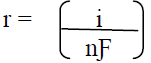(1)

where, again, n is the number of electrons associated with the ionization of each metal atom, and is 96,500 C/mol.

The corrosion rate of Al-Mn alloy in atmospheric environment has been predicted  based on direct input of initial weights of the alloy and its exposure times. The validity of the two-factorial polynomial derived model;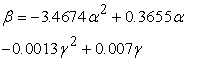(2)

is rooted on the core expression 0.2884 β = - α2 + 0.1054α – 3.7489 x 10-4 γ2 + 2.0186 x 10-3γ – 8.9396 x 10-4 where both sides of the expression are correspondingly approximately equal. Statistical analysis of model-predicted and experimentally evaluated corrosion rates as well as depth of corrosion penetration for each value of alloy initial weight and exposure time considered show standard errors of 0.0014 and 0.0015 % as well as 9.48 x10-4 and 8.64 x10-4 %, respectively. Corrosion rate per unit initial weight of exposed alloy as predicted by derived model and obtained from experiment are 1.8421 and 1.6316 (mm/yr) kg-1 respectively. Similarly, between exposure time: 0.0192 - 0.0628 yr, the depth of corrosion penetration on the exposed alloy as predicted by derived model and obtained from experiment are 1.5260 x 10-4 and 1.3516 x 10-4 mm respectively. Deviational analysis indicates that the maximum deviation of the model-predicted corrosion penetration rate from the corresponding experimental value is less than 11%.

Open system corrosion rate of aluminium-manganese alloy in sea water environment was assessed based on the alloy weight loss and exposure time . A model was derived and used as a tool for the assessment. It is made up of a quadratic and natural logarithmic function. The validity of the model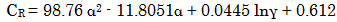(3)

is rooted on the core expression: 1.0126 x10-2 CR = α2 – 11.9538 x 10-2 α + 4.5059 x10-4 lnγ + 6.1968 x 10-3 where both sides of the expression are correspondingly approximately equal. Statistical analysis of model-predicted, regression-predicted and experimentally evaluated corrosion rates for each value of exposure time and alloy weight loss considered shows a standard error of 0.0657, 0.0709 & 0.0715 % and 0.0190 & 2.83 x 10-5 & 0.0068 % respectively. The resultant depth of corrosion penetration as predicted by derived model, regression model and obtained from experiment are 0.0102, 0.01 and 0.0112 mm respectively, while the corrosion rate per unit weight loss of the alloy as predicted by derived model, regression model and obtained from experiment are 7.7830, 7.6774 and 8.5777 mm/yr/g respectively. The maximum deviation of the model-predicted alloy corrosion rates from the corresponding experimental values is less than 27%.

Successful attempt  has been made to predict the possible exposure time for aluminium-manganese alloy (having particular corrosion rates and as-cast weights) in sea water environment. An empirical model was derived, validated and used for the analysis. The validity of the derived model;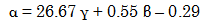(4)

is rooted on the core expression: 0.0375 α = γ + 0.0206 β – 0.0109 where both sides of the expression are correspondingly approximately equal. Statistical analysis of model-predicted and experimentally evaluated exposure time for each value of as-cast weight and alloy corrosion rate considered shows a standard error of 0.0017 & 0.0044 % and 0.0140 & 0.0150 % respectively. The depth of corrosion penetration (at increasing corrosion rate: 0.0104-0.0157 mm/yr) as predicted by derived model and obtained from experiment are 0.7208 x 10-4 & 1.0123 x 10-4 mm and 2.5460 x 10-4 & 1.8240 x 10-4 mm (at decreasing corrosion rate: 0.0157-0.0062 mm/yr) respectively. Deviational analysis indicates that the maximum deviation of the model-predicted alloy exposure time from the corresponding experimental value is less than 10%.

This research is aimed at carrying out an implicit analysis of aluminium-manganese alloy corrosion rate dependence on its pre-installed weight and exposure time in atmosphere environment. The difference between the present and past research  is just the role played by both the alloy pre-installed weight and its exposure time. In the previous research, both the pre-installed weight and exposure time played direct and separate roles, in that each was separately and directly used in the derived model to predict the corrosion rate. In the present research, both parameters will be used as an input ratio; weight/time to represent a single process parameter.

### 2. Materials and Methods

Materials used for the experiment are virgin aluminium of 99% purity and pure granulated manganese. The other materials used were acetone, sodium chloride, distilled water, beakers and measuring cylinders. The equipment used were lathe machine, drilling machine, crucible furnace, analytical digital weighing machine, reagents for etching and metallurgical microscope.

2.1. Specimen Preparation and Experimentation

Computation for each of the Al-Mn alloy compositions was carefully worked out, and the alloying materials charged into the surface crucible furnace. The molten alloy was cast into rods and allowed to cool in air (at room temperature). The cooled rods were machined to specific dimensions, cut into test samples and weighed. Each sample coupon was drilled with 5mm drill bit to provide hole for the suspension of the strings. The surface of each of the test coupons was thoroughly polished with emery cloth according to ASTM standards.

The method adopted for this phase of the research is the weight loss technique. The test coupons were exposed to the atmosphere environment and withdrawn after a known period of time. The withdrawn coupons were washed with distilled water, cleaned with acetone and dried in open air before weighing to determine the final weight.

### 3. Results and Discussion

Table 1 and Table 2 show that the corrosion rate of Al-Mn alloy increases with increase in the alloy exposure time (up to 240 hrs) and pre-installed weight. Increased alloy exposure time ensures prolonged physico-chemical interactions between the Al-Mn alloy and corrosion-induced agents resident in the atmosphere. Increased pre-installed weight increased the alloy corrosion rate through greater metal/alloy removal per unit area following activities of the corrosion-induced aggressive species (resident in the atmosphere) on the alloy. Table 1 and Table 2 present similar results except the alloy exposure time conversion from hrs to yrs.

#### Table 1. Variation of corrosion rate ζ of Al-Mn alloy with its exposure time ɤand pre-installed weight ϑDownload asVeiw tableTables index

#### Table 2. Variation of corrosion rate ζ of Al-Mn alloy with its evaluated exposure time ɤ (in years) and pre-installed weight ϑDownload asVeiw tableTables index

Surface structural Analysis of Corroded Al-Mn Alloy

Figure 4(a) depicts an un-corroded Al-Mn alloy which serves as control alloy, since it was not exposed to the atmosphere environment. The surface structure of this alloy shows an ash coloured background; a little shift from the normal colour of aluminium, due to presence of micro quantity of manganese. Figure 4(a) also shows that the control alloy is devoid of oxide films which results from initial corrosion attack.Download asVeiw figureFigures index
Figure 1. Surface structure of Al-Mn alloy (a) control (b), (c) (d), (e) and (f) after exposure times 0.0192, 0.0213, 0.0236, 0.026 and 0.0274 yrs respectively. (x200)

Figure 1(b - f) show different levels of intensive corrosion attacks at different exposure times. The white patches observed in Figure 1(b – f) are strongly believed to be oxide films produced during the initial corrosion attack on the Al-Mn alloy. Furthermore, the greenish patches also observed in Figure 1(b – f) are suspected to have resulted from prolonged oxidation of the alloy matrix during increased duration of their exposures to the atmosphere environment.

Since aluminum and its alloys are highly corrosion resistant in many environments due its ability to passivate, and the initially formed film protective, it is suspected that the prolonged oxidation of the Al-Mn matix was due to periodic changes in the concentration of the active corrosive species (from the atmosphere) as the exposure time is prolonged. This implied that the prolonged corrosion attack (as exposure time increased) on the alloy occurred because the passivated material initially formed on the alloy reverted to an active state due to the alteration in the concentration of the active corrosive species. This is in accordance with past research .

3.1. Model Formulation
3.1.1. Boundary and Initial Conditions

Consider solid Al-Mn alloy exposed to atmosphere environment and interacting with some corrosion-induced agents. The atmosphere is assumed to be affected by unwanted dissolved gases. Range of exposed time considered: 0.0192 - 0.0274 yrs (≈ 168 - 240 hrs). Alloy pre-installed weight range considered: 0.0114- 0.0121 kg. The quantity and purity of aluminium used were 99 wt % and 99% respectively. Concentration of manganese addition: 1wt %.

The boundary conditions are: aerobic environment to enhance Al-Mn alloy oxidation (since the atmosphere contains oxygen. At the bottom of the exposed alloy, a zero gradient for the gas scalar are assumed. The exposed alloy is stationary. The sides of the solid are taken to be symmetries.

#### Table 3. Variation of Log ζ + 2.5941 with 2.4908 (ϑ/ɤ) - 4.3059 (ϑ/ɤ)2Download asVeiw tableTables index

Computational analysis of generated experimental data shown in Table 2, gave rise to Table 3 which indicate that;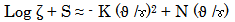(5)

Introducing the values of S, K and N into equation (5) reduces it to;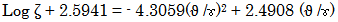(6)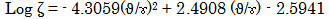(7)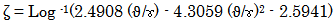(8)

Where

S = 2.5941, K = 4.3059 and N = 2.4908 are empirical constants (determined using C-NIKBRAN 

(ϑ) = Pre-installed weight,WG (kg)

(ɤ) = Exposure time, TM (yrs)

(ζ ) = Corrosion rate (mm/yr)

(ϑ/ɤ)= Input ratio WG/ TM (kg yr-1)

The derived model is equation (8). Computational analysis of Table 2 gave rise to Table 3. The derived model is two-factorial in nature since it is composed of two input process factors: alloy pre-installed weight and exposure time. This implies that the predicted corrosion rate of Al-Mn alloy in atmosphere environment is dependent on just two factors: pre-installed weight and exposure time

The validity of the model is strongly rooted on equation (6) (core model equation) where both sides of the equation are correspondingly approximately equal. Table 3 also agrees with equation (6) following the values of Log ζ + 2.5941 and - 4.3059(ϑ /ɤ)2 + 2.4908 (ϑ /ɤ) evaluated from the experimental results in Table 1. Furthermore, the derived model was validated by comparing the corrosion rate predicted by the model and that obtained from the experiment. This was done using various analytical techniques.

3.2. Statistical Analysis

Standard Error (STEYX)

The standard errors incurred in predicting Al-Mn corrosion rate for each value of WG, TM & WG/TM considered as obtained from experiment and derived model were 8.2146 x 10-5, 5.4602 x 10-5 & 1.22 x 10-4 % and 9.9081 x 10-5, 1.4 x 10-4 & 4.4653 x 10-5 % respectively. The standard error was evaluated using Microsoft Excel version 2003.

Correlation

The correlation coefficient between Al-Mn corrosion rate and WG, TM & WG/ TM were evaluated (using Microsoft Excel Version 2003) from results of the experiment and derived model. These evaluations were based on the coefficients of determination R2 shown in Figure 2-Figure 7.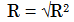(9)Download asVeiw figureFigures index
Figure 2. Coefficient of determination between Al-Mn corrosion rate and alloy exposure time as obtained from the experimentDownload asVeiw figureFigures index
Figure 3. Coefficient of determination between Al-Mn corrosion rate and alloy exposure tme as predicted by derived modelDownload asVeiw figureFigures index
Figure 4. Coefficient of determination between Al-Mn corrosion rate and alloy pre-installed weight as predicted by derived modelDownload asVeiw figureFigures index
Figure 5. Coefficient of determination between Al-Mn corrosion rate and alloy pre-installed weight as obtained from the experimentDownload asVeiw figureFigures index
Figure 6. Coefficient of determination between Al-Mn corrosion rate and input ratio WG/ TM as evaluated from experiment

#### Table 4. Comparison of the correlations between corrosion rate and exposure time as evaluated from experimental (ExD) and derived model (MoD) predicted resultsDownload asVeiw tableTables index

The evaluated correlations are shown in Table 4-Table 6. These evaluated results indicate that the derived model predictions are significantly reliable and hence valid considering its proximate agreement with results from actual experiment.Download asVeiw figureFigures index
Figure 7. Coefficient of determination between Al-Mn corrosion rate and input ratio WG/ TM as predicted by derived model

#### Table 5. Comparison of the correlations between corrosion rate and pre-installed weight and as evaluated from experimental and derived model predicted resultsDownload asVeiw tableTables index

#### Table 6. Comparison of the correlations between corrosion rate and input ratio of pre-installed weight and exposure time as evaluated from experimental and derived model predicted resultsDownload asVeiw tableTables index
3.3. Graphical Analysis

Comparative graphical analysis of Figure 8-Figure 10 shows very close alignment of the curves from derived model and experiment. It is strongly believed that the degree of alignment of these curves is indicative of the proximate agreement between ExD and MoD predicted results.Download asVeiw figureFigures index
Figure 8. Comparison of the Al-Mn corrosion rate (relative to alloy exposure time) as obtained from experiment and derived modelDownload asVeiw figureFigures index
Figure 9. Comparison of the Al-Mn corrosion rate (relative to alloy pre-installed weight) as obtained from experiment and derived modelDownload asVeiw figureFigures index
Figure 10. Comparison of the corrosion rate (relative to input ratio WG/ TM) as obtained from experiment and derived model

3.3.1. Comparison of Derived Model with Standard Model

The validity of the derived model was also verified through application of the regression model (Reg) (Least Square Method using Excel version 2003) in predicting the trend of the experimental results. Comparative analysis of Figure 11-Figure 13 shows closely aligned curves of corrosion rates, which precisely translated into significantly similar trend of data point’s distribution for experimental (ExD), derived model (MoD) and regression model-predicted (ReG) results of corrosion rates.Download asVeiw figureFigures index
Figure 11. Comparison of the corrosion rate (relative to alloy weight loss) as obtained from experiment, derived model and regression model

Also, the calculated correlations (from Figure 11-Figure 13) between Al-Mn corrosion rates and TM, WG & WG/TM for results obtained from regression model were 0.9969, 0.9968 & 0.9969 respectively. These values are in proximate agreement with both experimental and derived model-predicted results. The standard errors incurred in predicting Al-Mn corrosion rates for each value of TM, WG & WG/TM considered as obtained from regression model were 5.1841 x 10-5, 2.0162 x 10-5 and 2.9680 x 10-5 % respectively.Download asVeiw figureFigures index
Figure 12. Comparison of the corrosion rate (relative to alloy pre-installed weight) as obtained from experiment, derived model and regression modelDownload asVeiw figureFigures index
Figure 13. Comparison of the corrosion rate (relative to input ratio WG/ TM) as obtained from experiment, derived model and regression model
3.4. Computational Analysis

Computational analysis of the experimental and model-predicted corrosion penetration depth was carried out to ascertain the degree of validity of the derived model. This was done by comparing the depth of corrosion penetration obtained by calculation, using experimental and model-predicted results.

The depth of corrosion penetration on Al-Mn alloy during the period of exposure in the atmosphere environment

ζ D (mm) was calculated from the equation;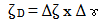(10)

Where

Δζ = Change in the corrosion rates (ζ 2 - ζ 1 ) within a range of exposure time: ɤ 1 - ɤ 2.

Δɤ = Change in the alloy exposure time ɤ 2, ɤ 1

Considering the points (0.0024, 0.0192) & (0.0041, 0.0274), (0.0023, 0.0192) & (0.0046, 0.0274) and (0.0023,0.0192) & (0.0040, 0.0274) as shown in Figure 11, then designating them as (ζ1, (ϑ /ɤ) 1) & (ζ2, (ϑ /ɤ) 2) for experimental, derived model and regression model predicted results respectively, and also substituting them into equation (10), gives the slopes: 1.394 x 10-5, 1.886 x 10-5 and 1.394 x 10-5 mm as their corrosion penetration depths respectively.

3.5. Deviational Analysis

Analysis of the corrosion rates precisely obtained from experiment and derived model shows deviations on the part of the model-predicted values relative to values obtained from the experiment. This is attributed to the fact that the surface properties of the Al-Mn alloy which played vital roles during the corrosion process were not considered during the model formulation. This necessitated the introduction of correction factor, to bring the model-predicted corrosion rate to those of the corresponding experimental values.

The deviation Dv, of model-predicted corrosion rate from the corresponding experimental result was given by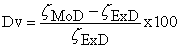(11)

Where

ζExD and ζMoD are corrosion rates evaluated from experiment and derived model respectively.

The correction factor took care of the negligence of operational contributions of surface properties of the Al-Mn alloy which actually affected the corrosion process. The model predicted results deviated from those of the experiment because these contributions were not considered during the model formulation. Introduction of the corresponding values of Cf from equation (12) into the model gives exactly the corresponding experimental corrosion rate.

#### Table 7. Variation of deviation and correction factor with input ratio WG /TMDownload asVeiw tableTables index

Comparative analysis of Figure 13 and Table 7 show that the maximum deviation of model-predicted corrosion rate from the experimental results was less than 19%. This translated into over 80% operational confidence and response level for the derived model as well as over 0.8 response coefficient of corrosion rate to the collective operational contributions of pre-installed alloy weight and exposure time in the atmosphere environment.

Table 7 and critical analysis of Figure 13 show that the least and highest magnitudes of deviation of the model-predicted corrosion rate (from the corresponding experimental values) are – 4.17 and + 18.75%. Figure 13 indicate that these deviations correspond to corrosion rates: 0.0023 and 0.0038 mm/yr, alloy pre-installed weight, WG: 0.0114 and 0.0118 kg, alloy exposure time, TM: 0.0192 and 0.0236 yrs as well as input ratio WG/ TM : 0.5938 and 0.5 kg yr-1 respectively.

Correction factor, Cf to the model-predicted results was given by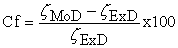(12)

Analysis of Table 7 also indicates that the evaluated correction factors are negative of the deviation as shown in equations (11) and (12).

Table 7 shows that the least and highest magnitudes of correction factor to the model-predicted corrosion rate are + 4.17 and - 18.75%. Figure 13 indicates that these deviations correspond to corrosion rates: 0.0023 and 0.0038 mm/yr, alloy pre-installed weight, WG: 0.0114 and 0.0118 kg, alloy exposure time, TM: 0.0192 and 0.0236 yrs as well as input ratio WG/ TM : 0.5938 and 0.5 kg yr-1 respectively.

It is important to state that the deviation of model predicted results from that of the experiment is just the magnitude of the value. The associated sign preceding the value signifies that the deviation is a deficit (negative sign) or surplus (positive sign).

### 4. Conclusion

Following an implicit analysis of the Al-Mn corrosion rate dependence on the pre-installed alloy weight and exposure time in atmosphere environment, surface structural analysis of the corroded alloy revealed in all cases widely distributed oxide film of the alloy in whitish form. A two-factorial empirical model was derived, validated and used for analysis of the highlighted dependence. The validity of the derived model was rooted on the core model expression Log ζ + 2.5941 = 2.4908 (ϑ/ɤ) - 4.3059 (ϑ/ɤ)2 where both sides of the expression are correspondingly approximately equal. Evaluations from generated results indicated that the corrosion penetration depth as obtained from experiment, derived model & regression model were 1.394 x 10-5, 1.886 x 10-5 & 1.394 x 10-5 mm respectively. Standard errors incurred in predicting the corrosion rate for each value of the pre-installed alloy weight and exposure time considered as obtained from experiment, derived model & regression model were 8.21 x 10-5, 9.91 x 10-5 & 2.02 x 10-5 % and 5.46 x 10-5, 1.4 x 10-4 & 5.18 x 10-5 % respectively. Deviational analysis indicates that the maximum deviation of model-predicted corrosion rate from the experimental results is less than 19%. This translated into over 80% operational confidence and response level for the derived model as well as over 0.8 response coefficient of corrosion rate to the collective operational contributions of pre-installed alloy weight and exposure time in the atmosphere environment.

### References

  Callister Jr, W. D. (2007). Materials Science and Engineering, 7th Edition, John Wiley & Sons Inc., USA.In article  Ekuma, C. E., and Idenyi, N. E. (2007). Statistical Analysis of the influence of Environment on Prediction of Corrosion from its Parameters. Res. J. Phy., USA, 1(1):27-34.In article  Stratmann, S. G., and Strekcel, H. (1990). On the Atmospheric Corrosion of Metals which are Covered with Thin Electrolyte Layers. II. Experimental Results. Corros. Sci.., 30:697-714.In article View Article  Polmear, I., J. (1981). Light Alloys. Edward Arnold Publishers Ltd.In article  Ekuma, C. E., Idenyi, N. E., and Umahi, A. E. (2007). The Effects of Zinc Addition on the Corrosion Susceptibility of Aluminium Alloys in Various Tetraoxosulphate (vi) Acid Environments. J. of Appl. Sci., 7(2):237-241.In article View Article  Nwoye, C. I., Idenyi, N. E., and Odo, J. U. (2012). Predictability of Corrosion Rates of Aluminum Manganese Alloys Based on Initial Weights and Exposure Time in the Atmosphere, Nigerian Journal of Materials Science and Engineering, 3(1):8-14.In article  Nwoye, C. I., Idenyi, N. E., Asuke, A. and Ameh, E. M. (2013). Open System Assessment of Corrosion Rate of Aluminum-Manganese Alloy in Sea Water Environment Based on Exposure Time and Alloy Weight Loss. J. Mater. Environ. Sci., 4(6): 943-952.In article  Nwoye, C. I., Neife, S., Ameh, E. M., Nwobasi, A. and Idenyi, N. E. (2013). Predictability of Al-Mn Alloy Exposure Time Based on Its As-Cast Weight and Corrosion Rate in Sea Water Environment. Journal of Minerals and Materials Characterization and Engineering, 1:307-314.In article View Article  Nwoye, C. I. (2008). C-NIKBRAN Data Analytical Memory (Software).In article PubMed